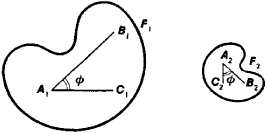# Similarity

(redirected from Similar (mathematics))
Also found in: Dictionary, Thesaurus.
The following article is from The Great Soviet Encyclopedia (1979). It might be outdated or ideologically biased.

## Similarity

a concept in geometry. Geometric figures are said to be similar if they are identical in shape, regardless of whether they are identical in size. The figures F1 and F2 are similar if between their points a one-to-one correspondence can be established such that the ratio of the distances between any two pairs of corresponding points of F1 and F2 a constant k. This constant is called the similarity factor. The angles between corresponding lines of similar figures are equal. Thus, in Figure 1 ∠B1A11C1 = ∠B2A2C2 = ϕ. The ratio of the areas of bounded similar figures is equal to the square of the similarity factor, and the ratio of the volumes of similar solids is equal to the cube of the similarity factor.Figure 1

A geometric transformation of the plane (or of space) such that all figures in the plane are transformed into similar figures with the same ratio of similitude is called a similarity transformation, which is a special case of an affine transformation. The set of all similarity transformations of the plane or of space forms a group. Any similarity transformation can be represented as the successive application of a homothetic transformation and a proper or improper motion.

The concept of similarity and similarity transformations are used in such engineering applications of geometry as modeling and drawing. For example, the operation of the pantograph is based on the concept of similarity.